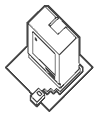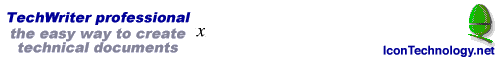15/4/99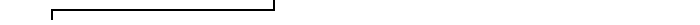## TechWriter professional

It's great! At the risk of writing advertising copy for Icon Technology, no piece of software on any platform has made me grin with delight as I discover new features as much as TechWriter has. Archive

## What is TechWriter? (read in conjunction with EasiWriter)

The flagship of the Icon range, TechWriter has all the features of its stable mate EasiWriter, with the addition of a powerful equation editor. It can be used as a basic word processor for writing short documents, yet has the flexibility to produce complete books or multi-column layouts incorporating equations, footnotes or endnotes and pictures created by other applications.

## Greek and symbols palette

Click on a character in the Greek and Symbols palette and it will be inserted into the document.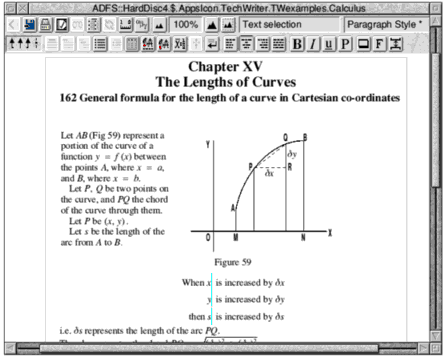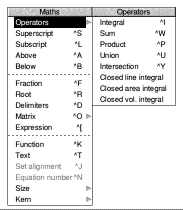## Greek characters and symbols

TechWriter comes complete with the MathPhys font:## Operators & Delimiters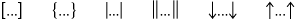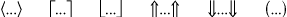## See how easy it is to create a formula

'...the output of mathematical formulae I've got with TechWriter is excellent and the program is so easy to use - congratulations.' Norbert Hauf - Germany.

 You type TechWriter displays A=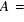TechWriter automatically italicises the 'A' and inserts the correct spacing between the characters. Ctrl-FThe fraction bar is drawn and the caret moves above it, centred, ready for you to type the numerator. 1, Return, n, Return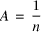Pressing Return moves the caret from numerator to denominator. The 'n' was automatically italicised. Ctrl-D, {TechWriter draws both brackets and positions the caret between them ready for you to type the contents. Note that delimiters grow automatically with their contents. Ctrl-W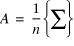TechWriter draws the '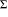' and positions the caret centrally beneath it, ready for you to type the lower limit. k=1, Return,nThe limits are automatically reduced in size and centred. Z, Ctrl-L, k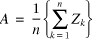TechWriter automatically reduces the size of the subscript. -BAlthough you type a dash TechWriter automatically substitutes a minus sign. It also inserts the correct spacing distinguishing betweenand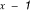.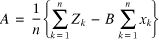Repeat steps 5, 6, 7 (typing 'x' instead of 'Z'.

A brilliant and exciting piece of software to use. The ease by which one can type complex mathematical expressions is remarkable. - Nigel Kirby

## HTML output

It can also create HTML files automatically converting equations into GIFs using !InterGif.

## TeX output

TechWriter Professional incorporates all the features of EasiWriter, EasiWriter Professional and TechWriter with the additional ability to save a document as a TeX file.## Part of the TeX output generated by TechWriter.

{\bf Example equations prepared using TechWriter on the Acorn Archimedes}\par\nobreak \fontsetab\vskip 10pt\leftskip=18pt\parfillskip=0pt plus1fil\spaceskip=0.3333em\xspaceskip=0.5em\relax Here is a short exploration of the mathematical typesetting abilities of TechWriter, beginning with an in-line quadraticequation: $ax^2+bx+c=0$ whose solution is given in the numberedand displayed equation that follows:$$\displayindent=18pt\displaywidth=445.4pt x={-b\pm\sqrt{b^2-4ac}\over2a}\eqno(1)$$One group of formul\ae\ which seem to defeat most systems arethose exponential equations like $v=V\left(1-e^{-{1\over CR}}\right)$ or $i=I_{\max}e^{-{Rt\over L}}.\,$ Now for some displayed statistics, with their summation signs and limits: $$\displayindent=18pt\displaywidth=445.4pt\bar x={x_1+x_2+x_3+\cdot\cdot\cdot+x_n\over n}={\sum^n_{i=0}x_i\over n}\eqno(2)$$$$\displayindent=18pt\displaywidth=445.4pt\sigma=\sqrt{\sum^n_{i =1}{\left(x_i-\bar x\right)}^{2^{}}\over n}\eqno(3)$$ Here is a series of aligned equations: \eqalignno{\int^{\pi\over4}_0\sin^2\theta\,{\rm d}\theta={}&{1\over 2}\int^{\pi\over4}_0(1-\cos2\theta){\rm d}\theta\cr\noalign{\vskip 4pt}={}&{1\over2}{\left[\theta-{1\over2}\sin2\theta\right]}^{\kern1pt{\pi\ov er4}}_{\kern1pt0}\cr\noalign{\vskip 2pt}={}&\left.{1\over2}\,[\left({\pi\over4}-{1\over2}\sin{\pi\over2}\right)- \left(0-{1\over2}\sin0\right)\right]\cr\noalign{\vskip 2pt}={}&{1\over2}\left[\left({\pi\over4}-{1\over2}\right)-(0)\right]\cr\noal ign{\vskip 2pt}={}&{1\over2}\left(\pi-2\over4\right)={\pi-2\over8}=0\cdot1427\cr}

## The TeX file displayed in a DVI viewer."TechWriter pro is the best damn word processor on *any* platform". Jim Lesurf on comp.sys.acorn.apps

A review of TechWriter by John Woodthorpe

Site map | About us | Products | Prices | Latest | Support

Top